# 18.9: Reactions at the $$\alpha$$ Carbons of Carboxylic Acid Derivatives

$$\newcommand{\vecs}{\overset { \rightharpoonup} {\mathbf{#1}} }$$ $$\newcommand{\vecd}{\overset{-\!-\!\rightharpoonup}{\vphantom{a}\smash {#1}}}$$$$\newcommand{\id}{\mathrm{id}}$$ $$\newcommand{\Span}{\mathrm{span}}$$ $$\newcommand{\kernel}{\mathrm{null}\,}$$ $$\newcommand{\range}{\mathrm{range}\,}$$ $$\newcommand{\RealPart}{\mathrm{Re}}$$ $$\newcommand{\ImaginaryPart}{\mathrm{Im}}$$ $$\newcommand{\Argument}{\mathrm{Arg}}$$ $$\newcommand{\norm}{\| #1 \|}$$ $$\newcommand{\inner}{\langle #1, #2 \rangle}$$ $$\newcommand{\Span}{\mathrm{span}}$$ $$\newcommand{\id}{\mathrm{id}}$$ $$\newcommand{\Span}{\mathrm{span}}$$ $$\newcommand{\kernel}{\mathrm{null}\,}$$ $$\newcommand{\range}{\mathrm{range}\,}$$ $$\newcommand{\RealPart}{\mathrm{Re}}$$ $$\newcommand{\ImaginaryPart}{\mathrm{Im}}$$ $$\newcommand{\Argument}{\mathrm{Arg}}$$ $$\newcommand{\norm}{\| #1 \|}$$ $$\newcommand{\inner}{\langle #1, #2 \rangle}$$ $$\newcommand{\Span}{\mathrm{span}}$$$$\newcommand{\AA}{\unicode[.8,0]{x212B}}$$

## The Acidic Properties of Esters with $$\alpha$$ Hydrogens

Many important synthetic reactions in which $$\ce{C-C}$$ bonds are formed involve esters and are brought about by basic reagents. This is possible because the $$\alpha$$ hydrogens of an ester, such as $$\ce{RCH_2CO_2C_2H_5}$$, are weakly acidic, and a strong base, such as sodium ethoxide, can produce a significant concentration of the ester anion at equilibrium: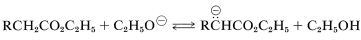The acidity of $$\alpha$$ hydrogens is attributed partly to the electron-attracting inductive effects of the ester oxygens, and partly to resonance stabilization of the resulting anion (Section 17-1A):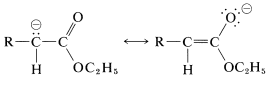When the $$\alpha$$ carbon of the ester carries a second strongly electron-attracting group, the acidity of $$\alpha$$ hydrogen is greatly enhanced. Examples of such compounds follow:The stabilization of the anions of these specially activated esters is greater than for simple esters because of the electron-withdrawing inductive effects of the substituents but more importantly because the negative charge can be distributed over more than two centers. Thus for the anion of ethyl 3-oxobutanoate we can regard all three of the valence-bond structures, $$14a$$ through $$14c$$, as important in contributing to the hybrid, $$14$$: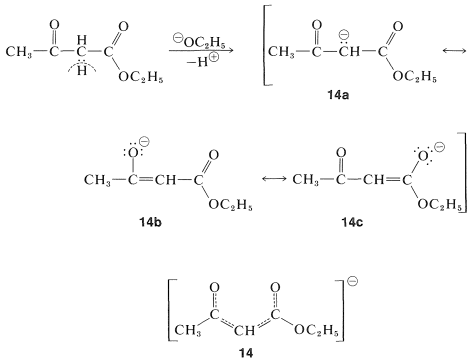The anion, $$14$$, is sufficiently stable relative to the ester that the $$K_\text{a}$$ is about $$10^{-11}$$ in water solution (Table 17-1).

Ethyl 3-oxobutanoate exists at room temperature as an equilibrium mixture of keto and enol tautomers in the ratio of 92.5 to 7.5. The presence of enol can be shown by rapid titration with bromine, but is more evident from the proton nmr spectrum (Figure 18-6), which shows absorption of the hydroxyl, alkenyl, and methyl protons of the enol form, in addition to absorptions expected for the keto form: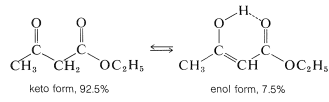Figure 18-6: Proton nmr spectrum of ethyl 3-oxobutanoate (ethyl acetoacetate) at $$60 \: \text{MHz}$$, calibrations are relative to tetramethylsilane at $$0.00 \: \text{ppm}$$. Peaks marked a, b, and c are assigned, respectively, to the $$\ce{OH}$$, alkenyl, and methyl protons of the enol form, whereas peaks d and e are assigned to the $$\alpha$$-$$\ce{CH_2}$$ and methyl protons, respectively, of the keto form. The quartet of lines at $$4.2 \: \text{ppm}$$ and the triplet at $$1.3 \: \text{ppm}$$ result from the ethyl groups of both keto and enol forms.

Interconversion of the enol and keto forms of ethyl 3-oxobutanoate is powerfully catalyzed by bases through the anion, $$15$$, and less so by acids through the conjugate acid of the keto form: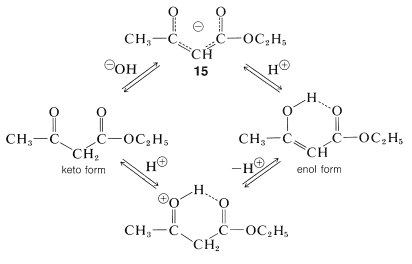Nonetheless, if contact with acidic and basic substances is rigidly excluded to the extent of using quartz equipment in place of glass (glass normally has a slightly alkaline surface), then interconversion is slow enough that it is possible to separate the lower-boiling enol from the keto form by fractional distillation under reduced pressure. The separated isomers are indefinitely stable when stored at $$-80^\text{o}$$ in quartz vessels.

## The Claisen Condensation

One of the most useful of the base-induced reactions of esters is illustrated by the self-condensation of ethyl ethanoate under the influence of sodium ethoxide to give ethyl 3-oxobutanoate:

$\ce{CH_3CO_2C_2H_5} + \ce{H-CH_2CO_2C_2H_5} \overset{^\ominus \ce{OC_2H_5}}{\longrightarrow} \ce{CH_3COCH_2CO_2C_2H_5} + \ce{C_2H_5OH}$

This reaction, called the Claisen condensation, is interesting because, from consideration of bond and stabilization energies, it is expected to be unfavorable thermodynamically with $$\Delta H^0$$ (vapor) equal to $$6 \: \text{kcal mol}^{-1}$$. This expectation is realized in practice, and much effort has been expended to determine conditions by which practical yields of the condensation product can be obtained.

The Claisen condensation resembles both the aldol addition (Section 17-3) and carbonyl additions of acid derivatives discussed previously (Sections 16-4 and 18-7). The first step, as shown in Equation 18-10, is the formation of the anion of ethyl ethanoate which, being a powerful nucleophile, attacks the carbonyl carbon of a second ester molecule (Equation 18-11). Elimination of ethoxide ion then leads to the $$\beta$$-keto ester, ethyl 3-oxobutanoate (Equation 18-12):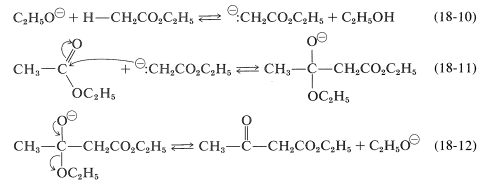The sum of these steps represents an unfavorable equilibrium, and satisfactory yields of the $$\beta$$-keto ester are obtained only if the equilibrium can be shifted by removal of one of the products.

One simple way of doing this is to remove the ethanol by distillation as it is formed; however, this may be difficult to carry to completion and, in any case, is self-defeating if the starting ester is low-boiling. Alternatively, one can use a large excess of sodium ethoxide. This is helpful because ethanol is a weaker acid than the ester enol, and excess ethoxide shifts the equilibrium to the right through conversion of the $$\beta$$-keto ester to the enolate salt (Equation 18-13).Obviously, the condensation product must be recovered from the enol salt and isolated under conditions that avoid reversion to starting materials. The best procedure is to quench the reaction mixture by pouring it into an excess of cold dilute acid.

A limitation on the Claisen condensation is that although the starting ester need have only one $$\alpha$$ hydrogen for Reactions 18-10 through 18-12 to occur, two $$\alpha$$ hydrogens are necessary for a favorable equilibrium utilizing the ionization reaction of Equation 18-13. As a result, it is not surprising to find that ethyl 2-methylpropanoate fails to condense with itself in the presence of sodium ethoxide, because the condensation product has no $$\alpha$$ hydrogen next to the ester group:However, it an excess of a very much stronger base than sodium ethoxide is used [such as triphenylmethylsodium, $$\ce{(C_6H_5)_3C}^\ominus \ce{Na}^\oplus$$], this same condensation does take place in reasonable yields. The reason is that the base is now strong enough to convert the alcohol formed in the reaction to sodium ethoxide, thus shifting the equilibrium to the right:The overall reaction then isClaisen condensations can be carried out between two different esters but, because there are four possible products, mixtures often result. Less difficulty is encountered if one of the esters has no $$\alpha$$ hydrogen and reacts readily with a carbanion according to Equations 18-11 and 18-12. The reaction then has considerable resemblance to the mixed aldol additions discussed in Section 17-3C. Among the useful esters without $$\alpha$$ hydrogens, and with the requisite electrophile reactivity, are those of benzenecarboxylic, methanoic, ethanedioic, and carbonic acids. Several practical examples of mixed Claisen condensations are shown in Equations 18-14 through 18-16 (all of the products exist to the extent of $$10\%$$ or so as the enol forms):An important variation on the Claisen condensation is to use a ketone as the anionic reagent. This often works well because ketones usually are more acidic than simple esters and the base-induced self-condensation of ketones (aldol addition) is thermodynamically unfavorable (Section 17-3C). A typical example is the condensation of cyclohexanone with diethyl ethanedioate (diethyl oxalate):$$\alpha$$-Keto esters of the type formed according to Equations 18-16 and 18-17 have synthetic utility in that they lose carbon monoxide when strongly heated:A somewhat similar decarbonylation reaction was mentioned previously for diphenylpropanetrione (Section 17-10).

## Alkylation of Ester Anions

The anions of esters such as ethyl 3-oxobutanoate and diethyl propanedioate can be alkylated with alkyl halides. These reactions are important for the synthesis of carboxylic acids and ketones and are similar in character to the alkylation of ketones discussed previously (Section 17-4A). The ester is converted by a strong base to the enolate anion, Equation 18-18, which then is alkylated in an $$S_\text{N}2$$ reaction with the alkyl halide, Equation 18-19. Usually, $$\ce{C}$$-alkylation predominates:Esters of propanedioic (malonic) acid can be alkylated in a similar fashion (Equation 18-20):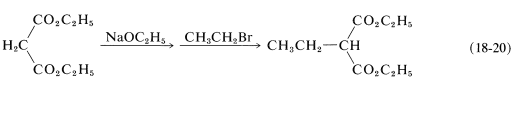Unfortunately, monoalkylation seldom occurs cleanly by the above sequence whenever the monoalkylation product has an $$\alpha$$ hydrogen located so as to permit dialkylation to occur. In practice, alkylation reactions, using one mole of ester, one mole of sodium ethoxide, and one mole of an alkyl halide (e.g., $$\ce{CH_3I}$$), give a mixture of the starting ester, its mono- and dialkylation products. The situation is more favorable when large alkyl groups are introduced, because then the physical properties and reactivities of the starting materials and of mono- and dialkylation products differ considerably. Usually dialkylation is inhibited by having a bulky alkyl group in the monoalkylation product.

Alkyl-substituted 3-oxobutanoic and propanedioic esters can be hydrolyzed under acidic conditions to the corresponding acids, and when these are heated they readily decarboxylate (Section 18-4). Alkyl 3-oxobutanoic esters thus yield methyl alkyl ketones, whereas alkylpropanedioic esters produce carboxylic acids:These reactions commonly are known as the acetoacetic-ester ketone and the malonic-ester acid syntheses, respectively.

Alkyl 3-oxobutanoic esters react with concentrated alkali by a different path to reverse the Claisen condensation: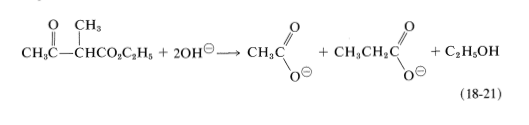## Acylation of Ester Anions

Enolate anions of esters, such as ethyl 3-oxobutanoate or diethyl propanedioate, react with acyl halides or anhydrides to give acylation products. These reactions are carried out best using sodium hydride instead of sodium ethoxide for production of the enol salt, because then no alcohol is liberated to react with the acyl halide or anhydride:## Aldol-Type Additions of Ester Anions and the Reformatsky Reaction

Addition of an ester anion to the carbonyl group of an aldehyde or ketone is a type of aldol addition (Section 17-3):There are certain difficulties in achieving this type of aldol reaction. First, alkali-induced ester hydrolysis would compete with addition. Second, a Claisen condensation of the ester might intervene, and third, the ester anion is a stronger base than the enolate anions of either aldehydes or ketones, which means reaction could be defeated by proton transfer of the typeHowever, a useful synthetic reaction can be achieved in the following way. First, the ester anion is formed in the absence of water without causing a Claisen condensation or other carbonyl addition. This can be done with ethyl ethanoate by treating it with lithium bis(trimethylsilyl)amide in oxacyclopentane solution at $$-80^\text{o}$$:The advantage of $$\ce{LiN[Si(CH_3)_3]_2}$$ as the base in this reaction is that $$\overset{\ominus}{\ce{N}} \ce{[Si(CH_3)_3]_2}$$ is a reasonably strong base; it is bulky, which inhibits addition to the carbonyl; and it also forms a weakly basic amine, $$\ce{HN[Si(CH_3)_3]_2}$$, which does not interfere in the subsequent reactions.

The solution of ethyl lithioethanoate must be kept cold and treated promptly with an aldehyde or ketone. Thus, with 2-propanone,For the reaction to be successful, the carbonyl addition has to be faster than the proton transfer reaction, $$\ce{LiCH_2CO_2C_2H_5} + \ce{CH_3COCH_3} \rightarrow \ce{CH_3CO_2C_2H_5} + \ce{LiCH_2COCH_3}$$ and, at $$-80^\text{o}$$, this is the case. This synthesis of $$\beta$$-hydroxy esters is a beautiful example of how rates of competing reactions can be manipulated to obtain a high yield of a desired addition product that may not be the most thermodynamically favorable one.

A closely related synthesis of $$\beta$$-hydroxy esters is provided by the Reformatsky reaction. This synthesis starts with an aldehyde or ketone, $$\ce{RCOR'}$$, and an $$\alpha$$-bromo ester, such as ethyl bromoethanoate. Zinc in a nonhydroxylic solvent (usually benzene) transforms the bromo ester into an organozinc compound, which then adds to the aldehyde or ketone carbonyl. Hydrolysis produces the $$\beta$$-hydroxy ester:As do aldols, $$\beta$$-hydroxy esters dehydrate (usually readily) to $$\alpha$$,$$\beta$$-unsaturated carbonyl compounds.## Biological Claisen Condensations and Aldol Additions. Fatty Acid Metabolism

The overall results of a Claisen condensation is the transfer of an acyl group $$\left( \ce{RCO}- \right)$$ from one ester molecule to another:Figure 18-7. Although it is large and complex, the reactive part for our discussion here is the thiol $$\left( \ce{SH} \right)$$ group. The thioester equivalent of the Claisen condensation of Equation 18-24 is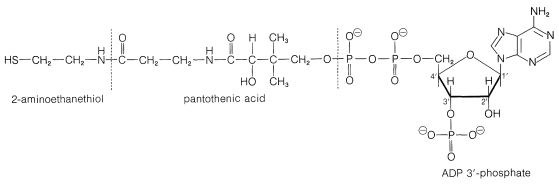Figure 18-7: The structure of coenzyme A ($$\ce{HS}$$CoA) showing the segments of which it can be considered to be constructed. The thiol group at the left end of the molecule reacts to form thioesters of the type $$\ce{RCOS}-$$CoA. The other parts of the coenzyme A molecule provide the structural elements that permit a high degree of specificity for interactions with enzymes.

The reverse of the above reaction is a key step in the oxidative degradation of fatty acids. This reverse Claisen condensation (catalyzed by thiolase) involves the cleavage of a carbon-carbon bond of a $$\beta$$-keto ester of coenzyme A by another molecule of coenzyme A to give a new acyl derivative ($$\ce{RCO-S}$$CoA) and ethanoyl (acetyl) derivative ($$\ce{CH_3O-S}$$CoA):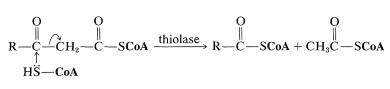Figure 18-8 and involve a sequence of enzymatic transformations of the typeFigure 18-8: Steps in the metabolic oxidation of a fatty acid. In each cycle of reactions, one mole of $$\ce{CH_3COS}$$CoA is formed and the alkyl group $$\ce{R}$$ is shortened by two carbons.

After formation of the $$\beta$$-keto thioester, it is cleaved by CoA$$\ce{SH}$$, and the resulting thioester goes back into the sequence two carbons shorter than before. In this way, a fatty acid is degraded from the carboxyl end, two carbons at a time.

There are two principle pathways for utilization of the ethanoyl coenzyme A ($$\ce{CH_3CO-S}$$CoA) formed in each turn of the oxidation cycle of Figure 18-8. Either it is used to synthesize larger molecules such as fatty acids, steroids, and so on, as will be described in Section 30-5A, or the acyl group is oxidized to $$\ce{CO_2}$$ and $$\ce{H_2O}$$:

$$\ce{CH_3CO-S}$$CoA $$\overset{\left[ \ce{O} \right]}{\rightarrow} 2 \ce{CO_2} + \ce{H_2O} + \ce{HS}$$CoA

The oxidation of the acyl group of coenzyme A is the net outcome of the citric acid or Krebs cycle (Section 20-10B). We will be interested here in the entry point of the cycle whereby ethanoyl coenzyme A is employed in a reaction that builds the $$\ce{C_6}$$ chain of citric acid (3-carboxy-3-hydroxypentanedioic acid) from $$\ce{C_2}$$ and $$\ce{C_4}$$ pieces: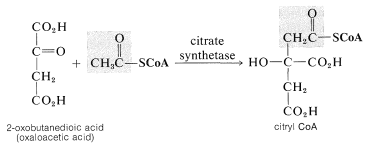This reaction is quite special in that it is an aldol-type addition in which a thioester is the donor (nucleophile) and a keto acid is the acceptor (electrophile). From the discussion in Section 18-8E, you will see that reactions of this kind involving an ester as the donor and an aldehyde or ketone as the acceptor can be achieved in the laboratory only under rather special conditions. For the thioester to function as a nucleophile at the $$\alpha$$ carbon under the restraints imposed by having the reaction occur at the physiological pH, the catalyzing enzyme almost certainly must promote formation of the enol form of the thioester. The enol then could add to the ketone carbonyl with the assistance of a basic group on the enzyme. This kind of catalysis by enzymes is discussed in Section 25-9C.$$^5$$Considerable confusion is possible because of the way in which biochemists use abbreviated names and formulas for the acyl derivatives of coenzyme A. To emphasize the vital $$\ce{-SH}$$ group, coenzyme A is usually written as CoA$$\ce{SH}$$. However, the acyl derivatives most often are called acetyl CoA and the like, not acetyl $$\ce{S}$$CoA, and you could well get the erroneous impression that the sulfur has somehow disappeared in forming the acyl derivative. We will include the sulfur in formulas such as $$\ce{CH_3COS}$$CoA, but use the customary names such as acetyl CoA without including the sulfur. To make clear that CoA does not contain cobalt, CoA is printed in this text in boldface type.

This page titled 18.9: Reactions at the $$\alpha$$ Carbons of Carboxylic Acid Derivatives is shared under a CC BY-NC-SA 4.0 license and was authored, remixed, and/or curated by John D. Roberts and Marjorie C. Caserio.